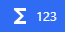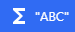# Expressions

When adding a condition or an action to an event, some fields have the following icons alongside them:These icons indicate that the field accepts an expression.

There are multiple types of expressions:

You can switch from Numbers to Text or Text to Numbers with an Functions.
For a complete list of the function expressions that GDevelop provides out of the box, refer to Expressions reference.

## Numbers

When the following icon appears alongside a field, the field accepts a numeric expression:A numeric expression is a number.

The following values are examples of numeric expressions:

• `0`
• `-10`
• `25.5`

You can also use mathematical operators to add, subtract, multiply, and divide numbers:

• `2+2`
• `3-1`
• `10*10`
• `99/3`

This is the complete list of operators:

• `+` (add)
• `-` (subtract)
• `*` (multiply)
• `/` (divide)
You can use multiple operators in a single expression.

## Text

When the following icon appears alongside a field, the field accepts a text expression:A text expression is a string of text.

The following values are examples of text expressions:

• `"Hello world"`
• `"This is a text expression"`
• `"GDevelop is cool!"`

All text expressions must be wrapped in double quotes. Without the double quotes, GDevelop assumes the value is a function, which will likely result in an error.

You can use the `+` operator to concatenate two or more strings of text. For example, `"Hello" + "World"` becomes `"HelloWorld"`. The other operators are not valid when working with text.

## Functions

Often, it's necessary to calculate complex values that mathematical operators can't handle. For example, if an event affects the position of the player, the game must dynamically calculate the X and Y coordinates of the player.

This is where functions come in.

You can use functions to dynamically calculate complex values while the game is running. For example, the `X` and `Y` functions calculate the coordinates of an object:

```ObjectName.X()
ObjectName.Y()```

Using a function is often referred to as calling a function.

If you're familiar with formulas in spreadsheets, functions in programming languages, or functions in math, know that functions in GDevelop behave in a similar way.

### Syntax

There are three types of functions:

• Functions without objects
• Functions with objects
• Functions with objects and behaviors

Each type of function has a (slightly) different syntax.

#### Functions without objects

Some functions exist independently of objects and behaviors. Your scene doesn't need to have any objects or behaviors to call these functions.

These are some examples of functions without objects:

• `CurrentSceneName()` - Get the name of the current scene.
• `FileSystem::DesktopPath()` - Get the path of the “Desktop” directory.
• `ToNumber(<number>)` - Convert a string into a number.
GDevelop uses the `::` syntax to namespace related functions. All of the file system functions, for instance, are prefixed with `FileSystem::`. This helps keep related functions organized. The `::` syntax doesn't affect the behavior of the function.

#### Functions with objects

Some functions are called on objects. This means the function affects or retrieves data about a specific object.

These are some examples of functions with objects:

• `<object>.Angle()` - Get the angle of the object (in degrees).
• `<object>.Layer()` - Get the name of the layer that the object is on.
• `<object>.ObjectName()` - Get the name of the object.

#### Functions with objects and behaviors

Some functions are called on an object's behavior. This means the function affects or retrieves data about a specific behavior that's attached to an object.

These are some examples of functions with objects and behaviors:

• `<object>.Pathfinding::Speed()`
• `<object>.Physics2::Friction()`
• `<object>.PlatformerObject::Gravity()`

### Arguments

Some functions accept arguments. An argument is a value that:

• can be passed into a function
• affects the output of the function

For example, the `ToString` function converts a number into a string, but it can't do anything unless you provide it with a number. That number is the argument. In the following example, the number `42` is the argument:

`ToNumber(42)`

Some functions, such as the `MouseX` function, accept multiple arguments:

`MouseX(layer, camera)`

When a function accepts multiple arguments, each argument must be separated by commas and provided in the specified order.

### Return values

When a function calculates a value, it's said to return that value. This value is the output of the function. For example, if the X coordinate of an object is `42`, the `ObjectName.X()` function returns `42`.

Functions can return numbers or strings. The type of value a function returns determines whether or not a function can be used in a field. For example, the `ToString` function returns a string, so it can't be used in a field that expects a number, while the `ToNumber` function returns a number, so it can't be used in a field that expects a string.